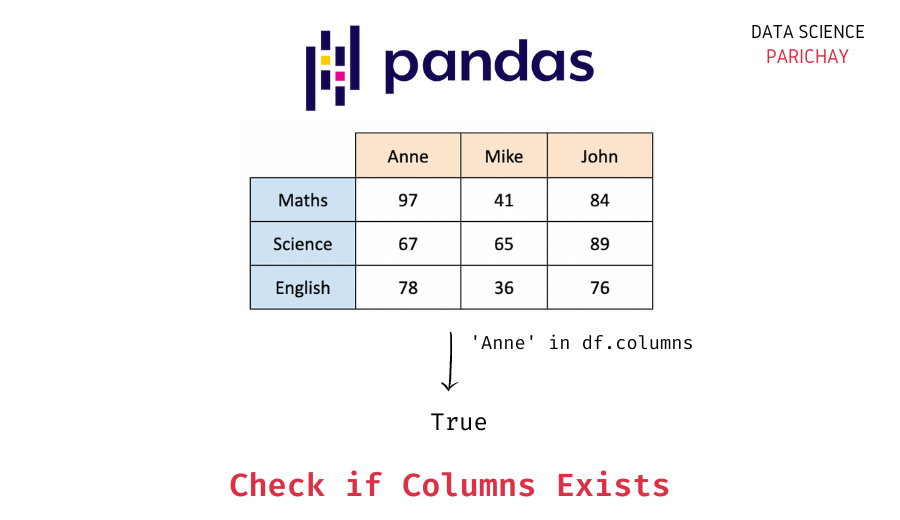# Check if a Column Exists in a Pandas DataFrame

In this tutorial, we will learn to check if a column exists in a Pandas DataFrame. A good level of exposure to Python is recommended but not required.

## How to check if a column exists in a dataframe?

The Pandas dataframe has a built-in method that returns its columns. Using this method, we can obtain the list of columns. We can then check if a column exists in the dataframe. The syntax will be-

`column_exists = column in df.columns`

Here,

• `df` — A Pandas DataFrame object.
• `df.columns` — Dataframe’s attribute that returns a list of columns as a Pandas Series object.

The `in` operator in Python is used to check if an item exists in the iterable over which it is applied. If the item exists, it returns `True`; otherwise, it returns `False`. Here, we have used the `in` operator to check if the column exists in the Series object returned by the `df.columns` attribute. Note that Series objects are iterable.

Another method to check if the column exists in the dataframe will be to try accessing the column. If the column exists, we can access the column; otherwise, an error will be raised. This error can be captured safely using a try-except block. The syntax for the same is-

```try:
try_accessing = df[column]
print(f"Column {column} exists in dataframe df")
except KeyError:
print(f"Column {column} doesn't exist in dataframe df")```

Here, the variables have the same meaning as in the previous code block. Under the `try` statement, we check if the column can be accessed. If it can’t be accessed, i.e., if the column doesn’t exist in dataframe `df`, `KeyError` is raised which is handled using `except` statement.

## Examples

Let’s understand the above code with some examples. To begin, we create a dataframe for the marks of students in a class. For simplicity, we will consider only three students and three subjects.

```import pandas as pd

#Making a dictionary to pass it to dataframe
d = {'Mary': [47, 25, 36], 'Alfred': [81, 85, 76], 'Jean': [93, 87, 71]}

#DataFrame object is created with index of choice.
df = pd.DataFrame(d, index=['Maths', 'English', 'Science'])

#Display the dataframe
print(df)```

Output:

📚 Data Science Programs By Skill Level

Introductory

Intermediate ⭐⭐⭐

🔎 Find Data Science Programs 👨‍💻 111,889 already enrolled

Disclaimer: Data Science Parichay is reader supported. When you purchase a course through a link on this site, we may earn a small commission at no additional cost to you. Earned commissions help support this website and its team of writers.

```         Mary  Alfred  Jean
Maths      47      81    93
English    25      85    87
Science    36      76    71```

We have the dataframe with subjects as row labels and student names as columns.

### Example 1: Check if the column exists using the `in` operator

To check if the column exists in the given dataframe, we print the result when the in operator is applied to the column and `df.columns`.

```column = 'Mary'
column_exists = column in df.columns
print(column_exists)```

Output:

`True`

The output is `True` since the column exists in the dataframe. If the column didn’t exist in the dataframe, the output would have been `False`.

### Example 2: Check if the column exists using try-except blocks

In this example, we will try to access the column of a dataframe under the `try` block. If it raises a `KeyError` while doing so, then the column doesn’t exist. Or else it exists. The code will be-

```column = 'Joseph'
try:
try_accessing = df[column]
print(f"Column {column!r} exists in dataframe df")
except KeyError:
print(f"Column {column!r} doesn't exist in dataframe df")```

Output:

`Column 'Joseph' doesn't exist in dataframe df`

Since the column ‘Joseph’ doesn’t exist, accessing the column in the `try` block raises a `KeyError`, and the code under the `except` block is executed. Note that `!r` is used only for formatting the output.

## Summary

From this tutorial, we looked at how to:

• Use the in operator and the columns method of Pandas DataFrame to check if the column exists in the dataframe.
• Use try-except blocks to check if the column exists in the dataframe.

You might also be interested in –

•